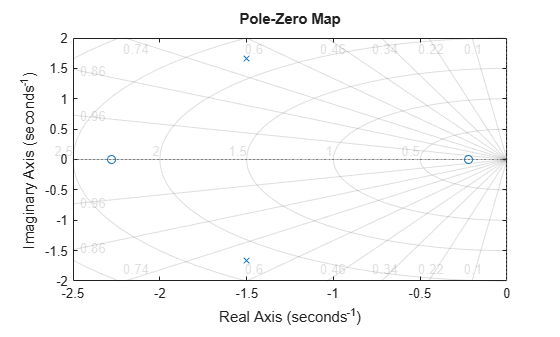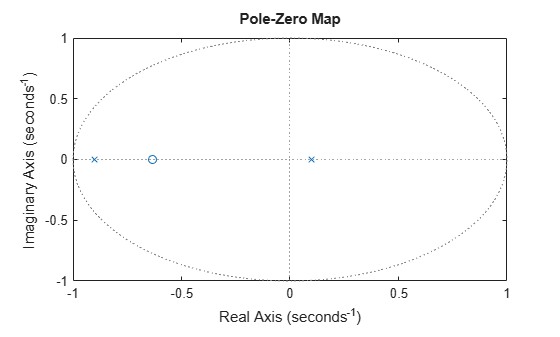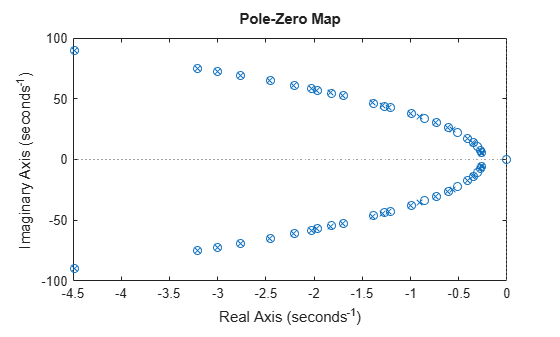Documentation

### This is machine translation

Mouseover text to see original. Click the button below to return to the English version of the page.

# pzmap

Pole-zero plot of dynamic system

## Syntax

``pzmap(sys)``
``pzmap(sys1,sys2,...,sysN)``
``[p,z] = pzmap(sys)``

## Description

example

````pzmap(sys)` creates a pole-zero plot of the continuous or discrete-time dynamic system model `sys`. `x` and `o` indicates the poles and zeros respectively, as shown in the following figure.From the figure above, an open-loop linear time-invariant system is stable if: In continuous-time, all the poles on the complex s-plane must be in the left-half plane (blue region) to ensure stability. The system is marginally stable if distinct poles lie on the imaginary axis, that is, the real parts of the poles are zero.In discrete-time, all the poles in the complex z-plane must lie inside the unit circle (blue region). The system is marginally stable if it has one or more poles lying on the unit circle. ```

example

````pzmap(sys1,sys2,...,sysN)` creates the pole-zero plot of multiple models on a single figure. The models can have different numbers of inputs and outputs and can be a mix of continuous and discrete systems. For SISO systems, `pzmap` plots the system poles and zeros. For MIMO systems, `pzmap` plots the system poles and transmission zeros.```

example

````[p,z] = pzmap(sys)` returns the system poles and transmission zeros as column vectors `p` and `z`. The pole-zero plot is not displayed on the screen.```

## Examples

collapse all

Plot the poles and zeros of the continuous-time system represented by the following transfer function:

`$H\left(s\right)=\frac{2{s}^{2}+5s+1}{{s}^{2}+3s+5}.$`

```H = tf([2 5 1],[1 3 5]); pzmap(H) grid on```Turning on the grid displays lines of constant damping ratio (zeta) and lines of constant natural frequency (wn). This system has two real zeros, marked by o on the plot. The system also has a pair of complex poles, marked by x.

Plot the pole-zero map of a discrete time identified state-space (`idss`) model. In practice you can obtain an `idss` model by estimation based on input-output measurements of a system. For this example, create one from state-space data.

```A = [0.1 0; 0.2 -0.9]; B = [.1 ; 0.1]; C = [10 5]; D = ; sys = idss(A,B,C,D,'Ts',0.1);```

Examine the pole-zero map.

`pzmap(sys)`System poles are marked by x, and zeros are marked by o.

For this example, load a 3-by-1 array of transfer function models.

```load('tfArray.mat','sys'); size(sys)```
```3x1 array of transfer functions. Each model has 1 outputs and 1 inputs. ```

Plot the poles and zeros of each model in the array with distinct colors. For this example, use red for the first model, green for the second and blue for the third model in the array.

```pzmap(sys(:,:,1),'r',sys(:,:,2),'g',sys(:,:,3),'b') sgrid````sgrid` plots lines of constant damping ratio and natural frequency in the s-plane of the pole-zero plot.

Use `pzmap` to calculate the poles and zeros of the following transfer function:

`$sys\left(s\right)=\frac{4.2{s}^{2}+0.25s-0.004}{{s}^{2}+9.6s+17}$`

```sys = tf([4.2,0.25,-0.004],[1,9.6,17]); [p,z] = pzmap(sys)```
```p = 2×1 -7.2576 -2.3424 ```
```z = 2×1 -0.0726 0.0131 ```

This example uses a model of a building with eight floors, each with three degrees of freedom: two displacements and one rotation. The I/O relationship for any one of these displacements is represented as a 48-state model, where each state represents a displacement or its rate of change (velocity).

```load('building.mat'); size(G)```
```State-space model with 1 outputs, 1 inputs, and 48 states. ```

Plot the poles and zeros of the system.

`pzmap(G)`From the plot, observe that there are numerous near-canceling pole-zero pairs that could be potentially eliminated to simplify the model, with no effect on the overall model response. `pzmap` is useful to visually identify such near-canceling pole-zero pairs to perform pole-zero simplification.

## Input Arguments

collapse all

Dynamic system, specified as a dynamic system model or model array. Dynamic systems that you can use include continuous-time or discrete-time numeric LTI models such as `tf`, `zpk`, or `ss` models.

If `sys` is an array of models, `pzmap` plots all the poles and zeros of every model in the array on the same plot.

## Output Arguments

collapse all

Poles of the system, returned as a column vector, in order of its increasing natural frequency. `p` is the same as the output of `pole(sys)`, except for the order.

Transmission zeros of the system, returned as a column vector. `z` is the same as the output of `tzero(sys)`.

## Tips

• Use the functions `sgrid` or `zgrid` to plot lines of constant damping ratio and natural frequency in the s- or z-plane on the pole-zero plot.

• For MIMO models, `pzmap` displays all system poles and transmission zeros on a single plot. To map poles and zeros for individual I/O pairs, use `iopzmap`.

• For additional options to customize the appearance of the pole-zero plot, use `pzplot`.